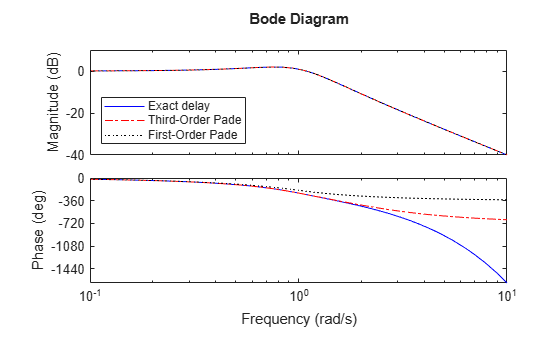Documentation

## Time-Delay Approximation in Continuous-Time Open-Loop Model

This example shows how to approximate delays in a continuous-time open-loop system using `pade`.

Padé approximation is helpful when using analysis or design tools that do not support time delays.

1. Create sample open-loop system with an output delay.```s = tf('s'); P = exp(-2.6*s)/(s^2+0.9*s+1);```

`P` is a second-order transfer function (`tf`) object with a time delay.

2. Compute the first-order Padé approximation of `P`.

`Pnd1 = pade(P,1)`
```Pnd1 = -s + 0.7692 ---------------------------------- s^3 + 1.669 s^2 + 1.692 s + 0.7692 Continuous-time transfer function. ```

This command replaces all time delays in `P` with a first-order approximation. Therefore, `Pnd1` is a third-order transfer function with no delays.

3. Compare the frequency response of the original and approximate models using `bodeplot`.

```h = bodeoptions; h.PhaseMatching = 'on'; bodeplot(P,'-b',Pnd1,'-.r',{0.1,10},h) legend('Exact delay','First-Order Pade','Location','SouthWest')```The magnitude of `P` and `Pnd1` match exactly. However, the phase of `Pnd1` deviates from the phase of `P` beyond approximately 1 rad/s.

4. Increase the Padé approximation order to extend the frequency band in which the phase approximation is good.

`Pnd3 = pade(P,3);`
5. Compare the frequency response of `P`, `Pnd1` and `Pnd3`.

```bodeplot(P,'-b',Pnd3,'-.r',Pnd1,':k',{0.1 10},h) legend('Exact delay','Third-Order Pade','First-Order Pade',... 'Location','SouthWest')```The phase approximation error is reduced by using a third-order Padé approximation.

6. Compare the time domain responses of the original and approximated systems using `stepplot`.

```stepplot(P,'-b',Pnd3,'-.r',Pnd1,':k') legend('Exact delay','Third-Order Pade','First-Order Pade',... 'Location','Southeast')```Using the Padé approximation introduces a nonminimum phase artifact (“wrong way” effect) in the initial transient response. The effect is quite pronounced in the first-order approximation, which dips significantly below zero before changing direction. The effect is reduced in the higher-order approximation, which far more closely matches the exact system’s response.

### Note

Using too high an approximation order may result in numerical issues and possibly unstable poles. Therefore, avoid Padé approximations with order N>10.